Search by Topic

Resources tagged with Interactivities similar to Amazing Alphabet Maze:

Filter by: Content type:
Age range:
Challenge level:

There are 141 results

Broad Topics > Information and Communications Technology > Interactivities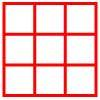Age 5 to 11 Challenge Level:

Place six toy ladybirds into the box so that there are two ladybirds in every column and every row.A Square of Numbers

Age 7 to 11 Challenge Level:

Can you put the numbers 1 to 8 into the circles so that the four calculations are correct?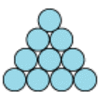Difference

Age 7 to 11 Challenge Level:

Place the numbers 1 to 10 in the circles so that each number is the difference between the two numbers just below it.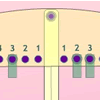Getting the Balance

Age 5 to 7 Challenge Level:

If you hang two weights on one side of this balance, in how many different ways can you hang three weights on the other side for it to be balanced?Junior Frogs

Age 5 to 11 Challenge Level:

Have a go at this well-known challenge. Can you swap the frogs and toads in as few slides and jumps as possible?One Million to Seven

Age 7 to 11 Challenge Level:

Start by putting one million (1 000 000) into the display of your calculator. Can you reduce this to 7 using just the 7 key and add, subtract, multiply, divide and equals as many times as you like?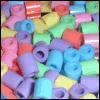Age 7 to 11 Challenge Level:

Three beads are threaded on a circular wire and are coloured either red or blue. Can you find all four different combinations?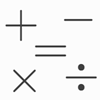Which Symbol?

Age 7 to 11 Challenge Level:

Choose a symbol to put into the number sentence.Age 7 to 11 Challenge Level:

If you have only four weights, where could you place them in order to balance this equaliser?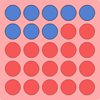All Change

Age 5 to 7 Challenge Level:

There are three versions of this challenge. The idea is to change the colour of all the spots on the grid. Can you do it in fewer throws of the dice?Times Tables Shifts

Age 7 to 11 Challenge Level:

In this activity, the computer chooses a times table and shifts it. Can you work out the table and the shift each time?Code Breaker

Age 7 to 11 Challenge Level:

This problem is based on a code using two different prime numbers less than 10. You'll need to multiply them together and shift the alphabet forwards by the result. Can you decipher the code?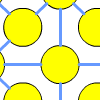One to Fifteen

Age 7 to 11 Challenge Level:

Can you put the numbers from 1 to 15 on the circles so that no consecutive numbers lie anywhere along a continuous straight line?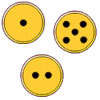Fair Exchange

Age 5 to 7 Challenge Level:

In your bank, you have three types of coins. The number of spots shows how much they are worth. Can you choose coins to exchange with the groups given to make the same total?Arrangements

Age 7 to 11 Challenge Level:

Is it possible to place 2 counters on the 3 by 3 grid so that there is an even number of counters in every row and every column? How about if you have 3 counters or 4 counters or....?More Transformations on a Pegboard

Age 7 to 11 Challenge Level:

Use the interactivity to find all the different right-angled triangles you can make by just moving one corner of the starting triangle.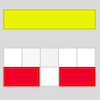Cuisenaire Counting

Age 5 to 7 Challenge Level:

Here are some rods that are different colours. How could I make a dark green rod using yellow and white rods?Domino Numbers

Age 7 to 11 Challenge Level:

Can you see why 2 by 2 could be 5? Can you predict what 2 by 10 will be?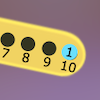Are You Well Balanced?

Age 5 to 7 Challenge Level:

Can you work out how to balance this equaliser? You can put more than one weight on a hook.Growing Garlic

Age 5 to 7 Challenge Level:

Ben and his mum are planting garlic. Use the interactivity to help you find out how many cloves of garlic they might have had.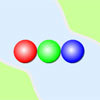Three Ball Line Up

Age 5 to 7 Challenge Level:

Use the interactivity to help get a feel for this problem and to find out all the possible ways the balls could land.Factor Lines

Age 7 to 14 Challenge Level:

Arrange the four number cards on the grid, according to the rules, to make a diagonal, vertical or horizontal line.Seven Flipped

Age 7 to 11 Challenge Level:

Investigate the smallest number of moves it takes to turn these mats upside-down if you can only turn exactly three at a time.Same Length Trains

Age 5 to 7 Challenge Level:

How many trains can you make which are the same length as Matt's, using rods that are identical?Making Trains

Age 5 to 7 Challenge Level:

Can you make a train the same length as Laura's but using three differently coloured rods? Is there only one way of doing it?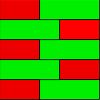Combining Cuisenaire

Age 7 to 11 Challenge Level:

Can you find all the different ways of lining up these Cuisenaire rods?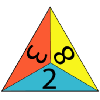One Big Triangle

Age 5 to 7 Challenge Level:

Make one big triangle so the numbers that touch on the small triangles add to 10.Multiples Grid

Age 7 to 11 Challenge Level:

What do the numbers shaded in blue on this hundred square have in common? What do you notice about the pink numbers? How about the shaded numbers in the other squares?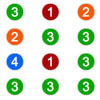Winning the Lottery

Age 7 to 11 Challenge Level:

Try out the lottery that is played in a far-away land. What is the chance of winning?Coded Hundred Square

Age 7 to 11 Challenge Level:

This 100 square jigsaw is written in code. It starts with 1 and ends with 100. Can you build it up?Countdown

Age 7 to 14 Challenge Level:

Here is a chance to play a version of the classic Countdown Game.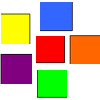Coloured Squares

Age 5 to 7 Challenge Level:

Use the clues to colour each square.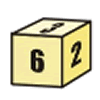First Connect Three for Two

Age 7 to 11 Challenge Level:

First Connect Three game for an adult and child. Use the dice numbers and either addition or subtraction to get three numbers in a straight line.Find the Difference

Age 5 to 7 Challenge Level:

Place the numbers 1 to 6 in the circles so that each number is the difference between the two numbers just below it.Teddy Town

Age 5 to 14 Challenge Level:

There are nine teddies in Teddy Town - three red, three blue and three yellow. There are also nine houses, three of each colour. Can you put them on the map of Teddy Town according to the rules?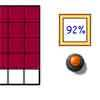100 Percent

Age 7 to 11 Challenge Level:

An interactive game for 1 person. You are given a rectangle with 50 squares on it. Roll the dice to get a percentage between 2 and 100. How many squares is this? Keep going until you get 100. . . .Building Stars

Age 7 to 11 Challenge Level:

An interactive activity for one to experiment with a tricky tessellation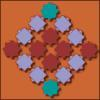Shapely Tiling

Age 7 to 11 Challenge Level:

Use the interactivity to make this Islamic star and cross design. Can you produce a tessellation of regular octagons with two different types of triangle?Counters

Age 7 to 11 Challenge Level:

Hover your mouse over the counters to see which ones will be removed. Click to remove them. The winner is the last one to remove a counter. How you can make sure you win?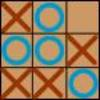Noughts and Crosses

Age 7 to 11 Challenge Level:

A game for 2 people that everybody knows. You can play with a friend or online. If you play correctly you never lose!Four Triangles Puzzle

Age 5 to 11 Challenge Level:

Cut four triangles from a square as shown in the picture. How many different shapes can you make by fitting the four triangles back together?Part the Piles

Age 7 to 11 Challenge Level:

Try to stop your opponent from being able to split the piles of counters into unequal numbers. Can you find a strategy?Colour Wheels

Age 7 to 11 Challenge Level:

Imagine a wheel with different markings painted on it at regular intervals. Can you predict the colour of the 18th mark? The 100th mark?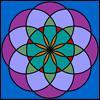Overlapping Circles

Age 7 to 11 Challenge Level:

What shaped overlaps can you make with two circles which are the same size? What shapes are 'left over'? What shapes can you make when the circles are different sizes?Two and One

Age 5 to 7 Challenge Level:

Terry and Ali are playing a game with three balls. Is it fair that Terry wins when the middle ball is red?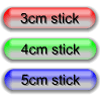Triangle Edges

Age 5 to 7 Challenge Level:

How many triangles can you make using sticks that are 3cm, 4cm and 5cm long?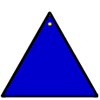Posting Triangles

Age 5 to 7 Challenge Level:

If you can post the triangle with either the blue or yellow colour face up, how many ways can it be posted altogether?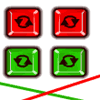Right Angle Challenge

Age 5 to 7 Challenge Level:

How many right angles can you make using two sticks?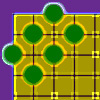Making Shapes

Age 5 to 7 Challenge Level:

Arrange any number of counters from these 18 on the grid to make a rectangle. What numbers of counters make rectangles? How many different rectangles can you make with each number of counters?Fractions and Coins Game

Age 7 to 11 Challenge Level:

Work out the fractions to match the cards with the same amount of money.